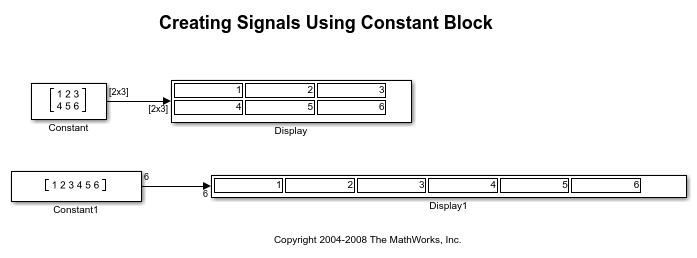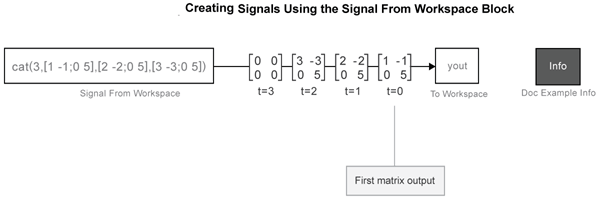## Create Signals for Sample-Based Processing

In sample-based processing, blocks process signals one sample at a time. Each element of the input signal represents one sample in a distinct channel. If the input signal is a matrix, each element corresponds to the same time. For example, from a sample-based processing perspective, this 3-by-2 matrix contains the first sample in each of the six independent channels.When you configure a block to perform sample-based processing, the block interprets a scalar input as a single-channel signal. Similarly, the block interprets an M-by-N matrix as a multichannel signal with M*N independent channels. For example, in sample-based processing, blocks interpret this sequence of 3-by-2 matrices as a six-channel signal.### Multichannel Signals for Sample-Based Processing

When you want to perform the same operations on several independent signals, you can group the signals into a multichannel signal. For example, if you need to filter each of four independent signals using the same direct-form II transpose filter, you can combine the signals into a multichannel signal, and connect the signal to a single Biquad Filter block. The block treats each element of the input as a channel when you set the Input processing parameter to ```Elements as channels (sample based)```. The block then applies the filter to each channel independently.

You can combine multiple independent signals into a single multichannel signal using the Concatenate (Simulink) block. You can also combine several multichannel signals into a single multichannel signal using the same technique.

This topic discusses creating signals for sample-based processing using the Constant block and the Signal From Workspace block. You can use a Matrix Concatenate (Simulink) block to combine these signals. The block receiving the signal implements sample-based processing or frame-based processing on the signal based on the Input processing parameter.

### Create Signals Using Constant Block

1. Create a new Simulink model.

2. From the Sources library, click and drag a Constant block into the model.

3. From the Sinks library, click and drag a Display (Simulink) block into the model.

4. Connect the two blocks.

5. Double-click the Constant block, and set the block parameters as follows:

• Constant value = [1 2 3; 4 5 6]

• Clear the Interpret vector parameters as 1-D parameter.

• Sample time = 1

Based on these settings, the Constant block outputs a constant, discrete-valued, 2-by-3 matrix signal with a sample period of 1 second.

You can set the Constant value parameter of the Constant block to any valid MATLAB variable or expression that evaluates to a matrix.

Save these parameters and close the dialog box by clicking OK.

In the Debug tab of the model toolstrip, under Information Overlays, click Signal Dimensions.

Run the model and expand the Display block so you can view the entire signal.

You have now successfully created a 2-by-3 signal with the sample period of 1 second.To view the model you just created, and to learn how to create a 1-D vector signal from the block diagram you just constructed, continue to the next section.

Create Unoriented Vector Signal

You can create an unoriented vector by modifying the block diagram you constructed in the previous section.

To add another signal to your model, copy the block diagram you created in the previous section and paste it below the existing signal in your model.

Double-click the Constant1 block, and set the block parameters as follows:

• Constant value = [1 2 3 4 5 6]

• Clear the Interpret vector parameters as 1-D parameter.

• Sample time = 1

Save these parameters and close the dialog box by clicking OK.

Run the model and expand the Display1 block so you can view the entire signal. Your model should now look similar to this figure. You can also open the equivalent model `ex_usingcnstblksb`.The Constant1 block generates a length-6 unoriented vector signal. This means that the output is not a matrix. However, most nonsource signal processing blocks interpret a length- M unoriented vector as an M-by-1 matrix (column vector).

### Create Signals Using Signal From Workspace Block

You can also use the Signal From Workspace block to create signals. In this section, the Signal From Workspace block creates a 2-by-2 signal with a sample period of 1 second.

1. Create a new Simulink model.

2. From the Sources library, click and drag a Signal From Workspace block into the model.

3. From the Simulink Sinks library, click and drag a To Workspace block into the model.

4. Connect the two blocks.

5. Double-click the Signal From Workspace block, and set the block parameters as follows:

• Signal = cat(3,[1 -1;0 5],[2 -2;0 5],[3 -3;0 5])

• Sample time = 1

• Samples per frame = 1

• Form output after final data value by = `Setting to zero`

Based on these settings, the Signal From Workspace block outputs a 2-by-2 signal with a sample period of 1 second. Once the block outputs the signal, all subsequent outputs have a value of zero.

Save these parameters and close the dialog box by clicking OK.

In the Debug tab of the model toolstrip, under Information Overlays, click Signal Dimensions.

Run the model.This figure is a graphical representation of the model behavior during simulation. You can also open the equivalent model `ex_usingsfwblksb`.At the MATLAB command line, type `yout`.

```yout = 1 -1 0 5 2 -2 0 5 3 -3 0 5 0 0 0 0 ```

You have now successfully created a 2-by-2 signal with sample period of 1 second using the Signal From Workspace block.

### Combine Signals Using Matrix Concatenate Block

You can combine individual signals into signals of larger dimensions using the Matrix Concatenate block.

In this example, the Matrix Concatenate block combines four 1-by-1 signals into a single 4-by-1 signal and two 4-by-1 signals into a single 4-by-2 signal.

Open and run the model.

In all the Signal From Workspace blocks in the model, the Sample time and the Samples per frame parameters are set to 1. The blocks output signals of size 1-by-1. The first two Matrix Concatenate blocks concatenate four 1-by-1 signals in the first dimension and output a 4-by-1 signal. The third Matrix Concatenate block concatenates the two 4-by-1 signals in the second dimension and outputs a signal of size 4-by-2.The To Workspace block in the model stores the 4-by-2 signal values at successive sample times in the `dsp_examples_yout` variable. You can see that the last matrix in `dsp_examples_yout` contains only zeros. This is because the Form output after final data value by parameter in all Signal From Workspace blocks in the model is set to `Setting to Zero`.

When you input the 4-by-2 signal to a block and set the Input processing parameter to `Elements as channels (sample based)`, the block performs sample-based processing. In the sample-based processing mode, the block treats each element as a channel, and the block processes the signal data one sample at a time.